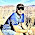## How to

When solving these problems, learning by doing is much better than learning by reading. I encourage to you read only as far in the solution as you need, then trying to solve the problem. If you get stuck, try reading a little further. And of course, let me know if you find a better solution!

## Wednesday, February 15, 2012

### Return Duplicated Number in an Array

Problem:  Given an array t with contains numbers between 1...98.  One value is duplicated -- return the duplicated values.  Try both O(n) and O(n-square).

int returnDuplicatedNum(int t[]){
...
}

Solution:
Let's start with a picture to make sure that we fully understand the problem.  Pic 1 is an example of this problem.  In Pic 1, we have one duplicated value, 18.

Well, let's think of how we can solve this problem.  The first way that might make sense is to do exactly what the problem asks -- start at the left hand side of the array, and iterate through.  For each value, check all the other values to the right and return true when we get to the end.  This will work correctly as we will eventually find the duplicated value if it's there.  This code is:

int returnDuplicatedNum(int t[]){

int i, j;

//iterate through the array
for(i=0; i < 100; i++) {
//now, check all the numbers to the right
//of the current value
for(j=i+1; j < 100; j++) {
//return the value if we find it duplicated
if(t[i]==t[j]) return t[i];
}
}
// no duplicate found
return -1;}

Well, what's the algorithmic efficiency of this solution?

Since, for each number, we check all the numbers to right, this would be an algorithm which wold be: n + (n-1) + (n-2)...  This is an O(n-squared solution).

This solution works, but it's relatively inefficient, or at least feels that.  Let's think of some alternatives.

Since we know that only one value is duplicated, we could order the array.  This would be an O(n log n) solution.  Then, we could iterate through the array and look for an element that had its duplicate next to it.  This would work, but would require reordering the elements in the array, which may not be desirable in some cases.

Also, let's look at some of the other information that we have on this array.  We know that all the numbers are between 1 and 99.  We essentially didn't use this information in either solution, as the numbers could have been anywhere in the integer set and our solution would work.  So, let's look at what relevant with this information.

First off, we know that if we had all the numbers between 1 and 99, we could easily sum them up.

Since only one element is duplicated, we know that if we sum up our array, we will get that sum + our duplicated element.  Now, we can use the difference between the expected sum and the actual sum to determine the duplicated element (some thought here to think about this).

Let's give some thought to how we can calculate the expected sum.  One way is to make a for loop and sum the elements, but this is essentially unnecessary.  If we think of the numbers between 1...98, we know that there are 49 sets of numbers which sum up to 99.  (Draw this out if it isn't clear, but essentially the ends of the array can come together.

So, the sum would be:  49 x 99.

So, knowing this, we can write our new code:

int returnDuplicatedNum(int t[]){

int i, sum;

for(i=0, sum=0; i < 100; i++)
sum+=t[i];

return(sum-(49*99));
}

Well, that's much more elegant.  And, since we're only iterating though the list once, this is O(n).  It's much more efficient than other solutions, and fairly elegant to code up.

This problem is a good example of where the first solution can often be found, but it doesn't use all of the information.  It these cases, it's often useful to go back to the original problem and see if it has more information in it that can be useful, such as the numbers in the array in this case, and then think about a clever way to use them.

/* Please let me know if you find alternative solutions, or bugs, or comments.  Thanks, Noah */

1.Another O(n) running time solution: If space isn't a constraint, you could also throw the values into a hash table and wait for a collision.

2.Good point. Thx Aniv.

3.Nit picking, but I believe there's an off-by-one error in the problem statement, you've specified t but I think you only need t. Numbers from 1..98 take up only t, so if you have a single duplicate number you will only need t.

There are some inconsistencies throughout the problem where you refer to the range being 1..99 instead: "..We know that all the numbers are between 1 and 99."